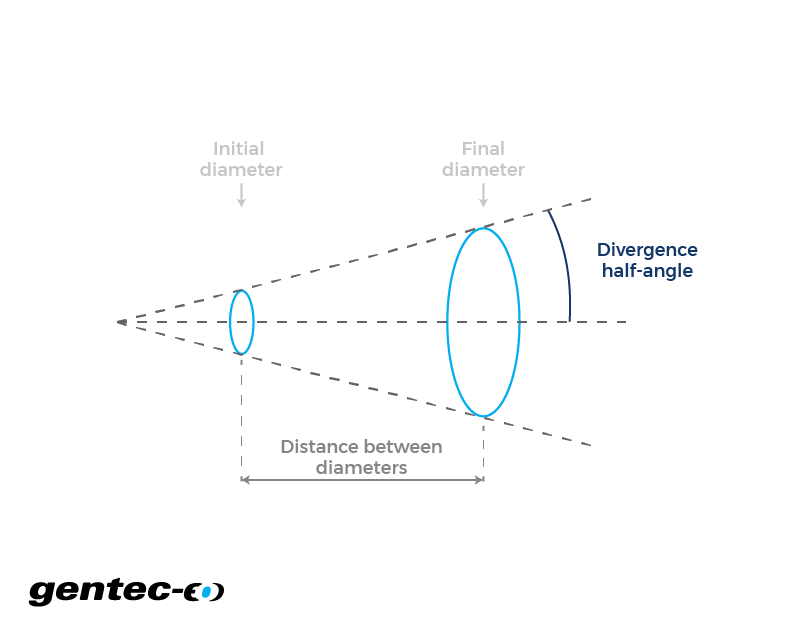EN

# Beam divergence and diameter

Calculate your laser beam divergence (half-angle) and diameter easily with this online calculator.

-

-

## How does the laser beam divergence and diameter calculator work?

In optics, laser beam divergence refers to how much a beam spreads over a distance. Imagine a cone where the tip is the theoretical origin of the laser beam (where the beam diameter would be punctual). If you measure the angle between two vectors, one going from the tip of the cone to the center of the base and the second going from the tip of the cone to the side of the base, the resulting angle is what you would call the divergence half-angle. Obviously, the full angle would be double that. Then, knowing three out of four parameters amongst initial diameter, final diameter, distance in between diameters and divergence angle, will give all the necessary information to calculate the fourth parameter. Also, if one understands the picture clearly, he will realize that the initial diameter can be, in fact, any diameter along the Z axis and doesn't need to be the one at the laser's exit.

Click to
enlarge## Formulas for laser beam diameter and divergence

As for all of our laser calculators, we assume that the beam is shaped as a perfect TEM₀₀ Gaussian beam and that the divergence is constant. This implies that we also assume that the two diameters are both inside the same homogenous, continuous and isotropic medium. Otherwise, divergence would be a function of space, therefore not constant. These equations can be used to calculate the laser beam diameters and divergence (half-angle).

Ref : Saleh, B. E. A., & Teich, M. C. (2007). Fundamentals of Photonics (2nd ed.). Wiley-Interscience.

$$\text{Divergence (half-angle)} = Arctan\left(\frac{\text{Second diameter}(m) - \text{First diameter}(m)}{2 \times \text{Distance between diameters}(m)}\right)$$

Right-click
to copy

$$\text{Beam diameter}(m) = 2 \times \text{Distance}(m) \times Tan(\text{Divergence(half-angle)}) + \text{Initial diameter}(m)$$

Right-click
to copy